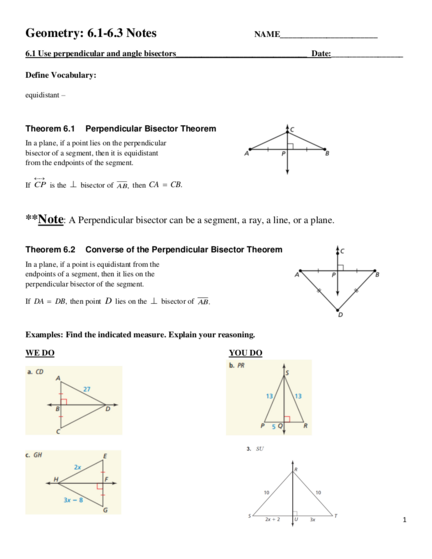# Using Angle Bisectors , Incenter, CircumcentreContributed by:This pdf includes the following topics:-
Perpendicular Bisector Theorem
Converse of the Perpendicular Bisector Theorem
Angle Bisector
Orthocenter
Centroid
1. Geometry: 6.1-6.3 Notes NAME_______________________
6.1 Use perpendicular and angle bisectors_______________________________ Date:_________________
Define Vocabulary:
equidistant –
Theorem 6.1 Perpendicular Bisector Theorem
In a plane, if a point lies on the perpendicular
bisector of a segment, then it is equidistant
from the endpoints of the segment.

If CP is the  bisector of AB, then CA  CB.
**Note: A Perpendicular bisector can be a segment, a ray, a line, or a plane.
Theorem 6.2 Converse of the Perpendicular Bisector Theorem
In a plane, if a point is equidistant from the
endpoints of a segment, then it lies on the
perpendicular bisector of the segment.
If DA  DB, then point D lies on the  bisector of AB.
Examples: Find the indicated measure. Explain your reasoning.
WE DO YOU DO
1
2. Examples: Find the indicated measure. Explain your reasoning.
WE DO YOU DO
2
3. Steps to find Perpendicular Bisector:
1. Find the midpoint of the segment
2. Find the slope of the segment
3. Then find the perpendicular slope.
4. Using the perpendicular slope and midpoint, find the equation of the perpendicular bisector.
Examples: Write an equation of a perpendicular bisector of the segment with the given endpoints.
WE DO YOU DO
D(5, -1) and E(-11, 3) A(0, -2) and B(2, 2)
Assignment
3
4. 6.2 Use bisectors of triangles___________________ ____________________________Date:_____________
Define Vocabulary:
concurrent –
point of concurrency –
circumcenter –
incenter –
4
5. Steps to find the Circumcenter:
1. Graph the triangle
2. Find the perpendicular bisectors of 2 sides (horizontal and vertical sides if possible).
3. Find the midpoint of the remaining side to verify the x-coordinate of the circumcenter.
Examples: Find the coordinates of the circumcenter of the triangle with the given vertices.
WE DO YOU DO
D(6, 4), E(-2, 4), F(-2, -2) R(0, 0), S(-4, 0), T(-6, 6)
Examples: N is the incenter of the triangle. Use the given information to find the indicated measure.
WE DO YOU DO
ND  2 x  5 NG  x  1
NE   2 x  7 NH  2 x  6
Find NF. Find NJ .
5
6. Examples: Find the indicated measure.
WE DO YOU DO
GE NF
PS PA
Assignment
6
7. 6.3 Using Medians and Altitudes of Triangles._________________________________Date:_____________
Define Vocabulary:
median of a triangle –
centroid –
altitude of a triangle –
orthocenter –
Theorem 6.7 Centroid Theorem
The centroid of a triangle is two-thirds of the distance from each
vertex to the midpoint of the opposite side.
The medians of ∆𝐴𝐵𝐶 meet at point P, and
2 2 2
AP  AE , BP  BF , and CP  CD.
3 3 3
Examples: Using the Centroid of a triangle.
WE DO YOU DO
Point P is the centroid. Find PN and QP.
QN  33
Point P is the centroid. Find PN and QP. Point D is the centroid. Find CD and CE.
7
8. Steps to find the Centroid of the triangle:
1. Graph the triangle
2. Find the midpoint of two of the sides
3. Then connect the midpoint with the opposite vertex of the triangle.
4. Repeat steps 2-3 for the remaining sides
5. Point of intersection is the centroid.
Examples: Find the coordinates of the centroid of the triangle with the given vertices.
WE DO YOU DO
A(0, 4), B(-4, -2), and C(7, 1) F(2, 5), G(4, 9) and H(6, 1)
8
9. Steps to find the Orthocenter of the triangle:
1. Graph the triangle.
2. Find the given slope and the perpendicular slope of a side
3. Draw the altitude of that line by starting at the opposite vertex and using the perpendicular slope
4. Repeat steps 2-3 to draw 2nd altitude. The point of intersection is the orthocenter.
Examples: Find the coordinates of the orthocenter of the triangle with the given vertices.
WE DO YOU DO
D(0, 6), E(-4, -2), and F(4, 6) J(-3, -4), K(-3, 4), and L(5, 4)
D(3, 4), E(11, 4), and F(9, -2)
Assignment
9